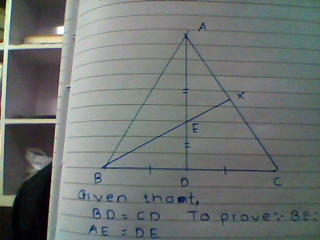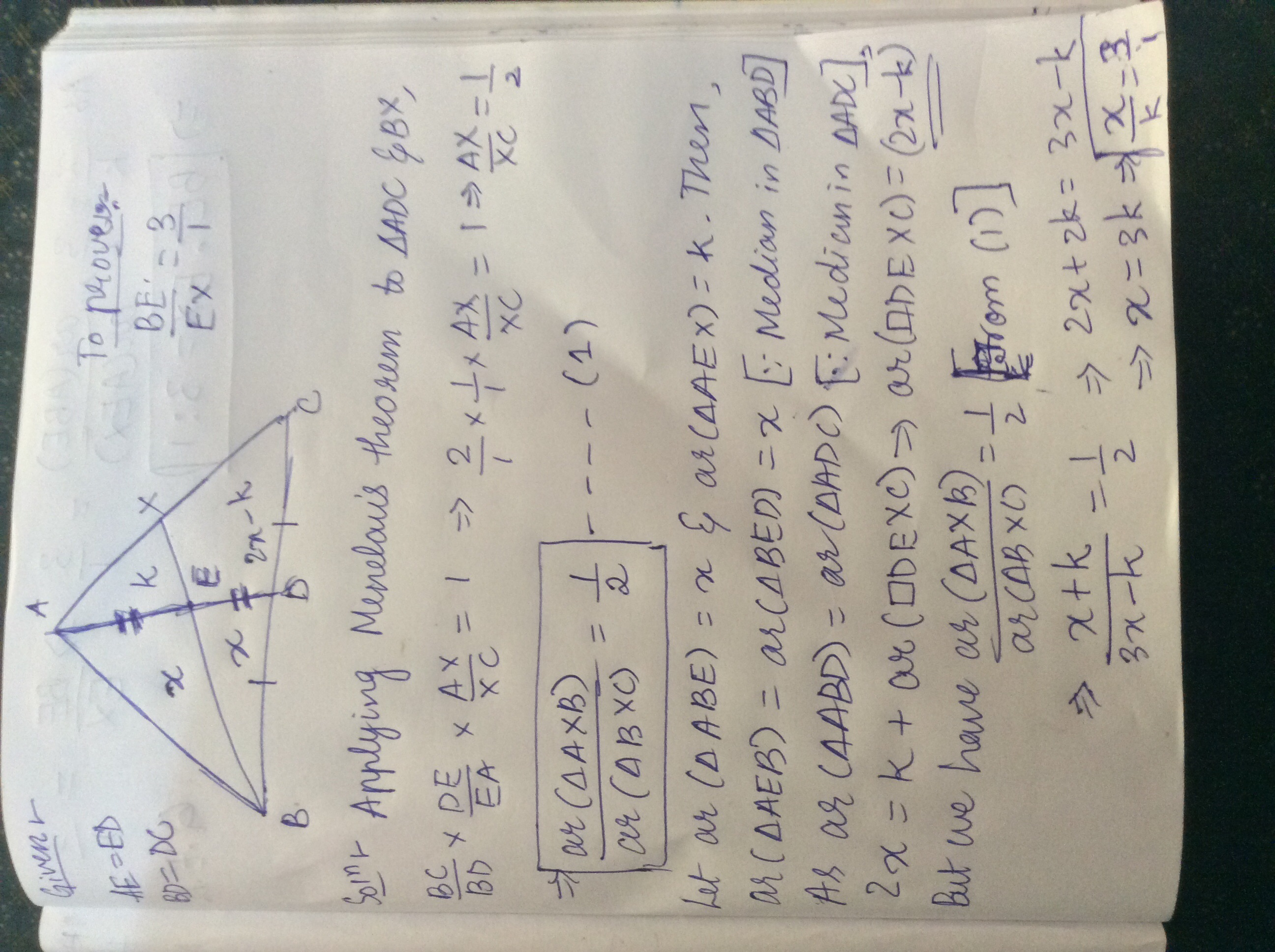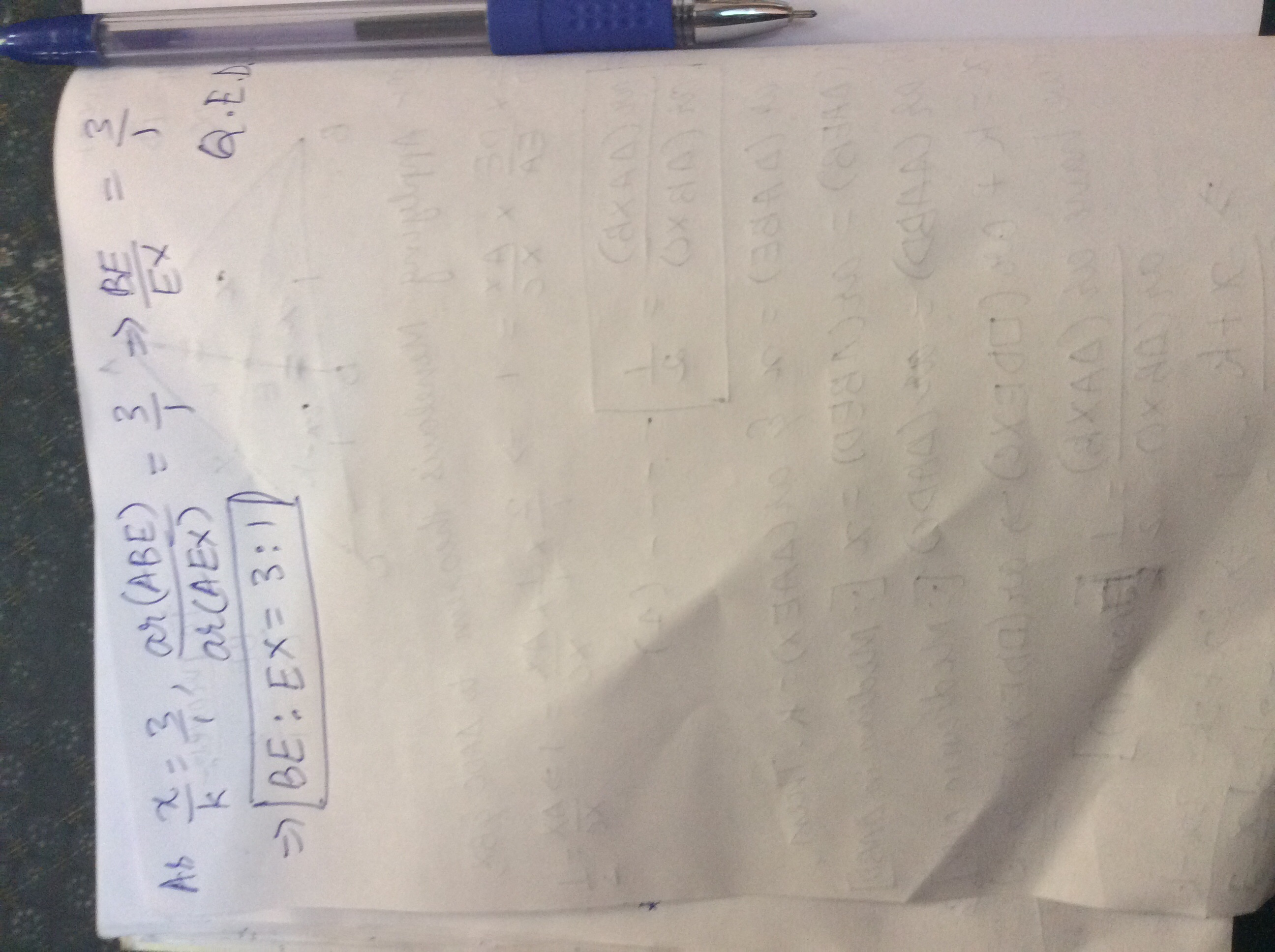# Think about triangles...You have to prove that BE:EX =3:1Note by A Former Brilliant Member
6 years ago

This discussion board is a place to discuss our Daily Challenges and the math and science related to those challenges. Explanations are more than just a solution — they should explain the steps and thinking strategies that you used to obtain the solution. Comments should further the discussion of math and science.

When posting on Brilliant:

• Use the emojis to react to an explanation, whether you're congratulating a job well done , or just really confused .
• Ask specific questions about the challenge or the steps in somebody's explanation. Well-posed questions can add a lot to the discussion, but posting "I don't understand!" doesn't help anyone.
• Try to contribute something new to the discussion, whether it is an extension, generalization or other idea related to the challenge.
• Stay on topic — we're all here to learn more about math and science, not to hear about your favorite get-rich-quick scheme or current world events.

MarkdownAppears as
*italics* or _italics_ italics
**bold** or __bold__ bold
- bulleted- list
• bulleted
• list
1. numbered2. list
1. numbered
2. list
Note: you must add a full line of space before and after lists for them to show up correctly
paragraph 1paragraph 2

paragraph 1

paragraph 2

[example link](https://brilliant.org)example link
> This is a quote
This is a quote
    # I indented these lines
# 4 spaces, and now they show
# up as a code block.

print "hello world"
# I indented these lines
# 4 spaces, and now they show
# up as a code block.

print "hello world"
MathAppears as
Remember to wrap math in $$ ... $$ or $ ... $ to ensure proper formatting.
2 \times 3 $2 \times 3$
2^{34} $2^{34}$
a_{i-1} $a_{i-1}$
\frac{2}{3} $\frac{2}{3}$
\sqrt{2} $\sqrt{2}$
\sum_{i=1}^3 $\sum_{i=1}^3$
\sin \theta $\sin \theta$
\boxed{123} $\boxed{123}$

## Comments

Sort by:

Top Newest

Just construct DF parallel to BX . So by MPT in BXC. DF = 1/2 BX. Now DF // EX. So by MPT in triangle ADF. We get XE = 1/2 DF. i.e. XE = 1/4 BX and so BE:EX = 3:1

- 6 years ago

Log in to reply

Nice solution :).

Log in to reply

You have Given that BD=CD and AE=DE.

Log in to reply

If you apply Menelaus' theorem twice you will get that ratio.

- 6 years ago

Log in to reply

can we do it by applying mid point theorem?

Log in to reply

I do not see how it could be helpful in this case.

- 6 years ago

Log in to reply

draw a line parallel to BC through the point E. say PQ,then in triangle ABD, E is mid point of AD and also,EP parallel to BD,therefore AP=BP,and similarly AQ=QC.Now Triangle BXC is similar to Triangle EXQ, Therefore,BX/EX=XC/XQ=BC/EQ.Let us take,BX/EX=BC/EQ,i.e BX/EX=BC/1/2DC, i.e BX/EX=BC/1/2(1/2B/C),That gives,BX/EX=4/1, Now subtracting 1 from both sides,that gives, BE:EX=3:1

I think Actually we can prove this by 3 ways.

Log in to reply

I am glad you have found your answers. As always in math, there are many ways to do things.

- 6 years ago

Log in to reply

Yeah thank you.

Log in to replyLog in to reply

yeah but we can also do it by the use of mid point theorem , just draw a line parallel to BC through point E.and further you can solve

Log in to reply

×

Problem Loading...

Note Loading...

Set Loading...